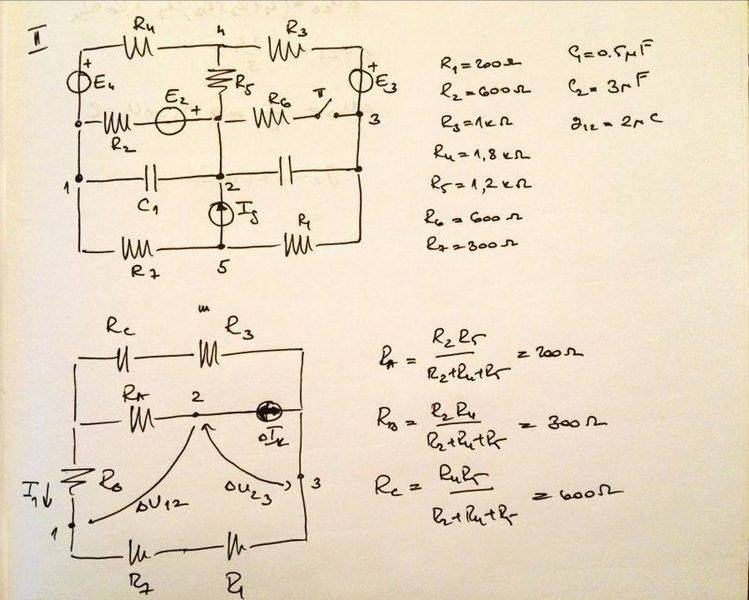# Transformation of resistors in a circuit

• Engineering

## Homework Statement

From the circuit diagram ( http://postimg.org/image/lldnr7mf7/ ) calculate the net charge flown through the capacitor 2.

## Homework Equations

3. The Attempt at a Solution [/B]
I actually don't need to solve the full problem as i understand how, what i have trouble with is the first part. Below the circuit diagram there is a transformation diagram where i suppose we eliminated every generator that stays the same throughout the changes in the circuit. (In the text we first had switch open and then it closes. ##q1## is the flow thorough the capacitor 1 but lets not dwell on that.
My real questions are:
Where did ##R_6## go? That side is where the switch is so i guess that its disclusion has something to do with that but why exactly?
What exactly is the rule for getting ##R_a## and ##R_c## and why is ##R_B## exactly there?
I see that they are all calculated similarly, product of two resistors divided by the total sum of resistors and the resultant are connected in parallel, is that right? Is there a simpler diagram you can refer me to which uses this?

gneill
Mentor
Can you upload an image to Physics Forums so we don't have to follow a link off-site to view it? For some reason I can't see the small version of the image on your image site, and can't pan the large version. It's better to upload here.

From what I can make out it's likely that a Δ-Y transform was performed on resistors R2, R4, and R5. Look up "Delta-Y transform". As for R6, if the reduced circuit pertains to the time when the switch is open, then the branch with the switch and R6 can be ignored as no current can flow through an open circuit.

Can you upload an image to Physics Forums so we don't have to follow a link off-site to view it? For some reason I can't see the small version of the image on your image site, and can't pan the large version. It's better to upload here.

From what I can make out it's likely that a Δ-Y transform was performed on resistors R2, R4, and R5. Look up "Delta-Y transform". As for R6, if the reduced circuit pertains to the time when the switch is open, then the branch with the switch and R6 can be ignored as no current can flow through an open circuit.
Sure, here it is:And here is the original text:
"The switch is open in state 1 and then it closes. During the time until the stationary condition appears, ##+2uC## of charge flows through the wire with ##C_1## Calculate the amount that goes through the wire with ##C_2##."
Using this transfiguration we have gotten the same answer as the book gives. (##-22uC##). What do you think happend with ##R_6##? Why doesnt it have a role in the new circuit?

gneill
Mentor
What do you think happend with ##R_6##? Why doesnt it have a role in the new circuit?
If the transformed circuit is meant to include the switch branch with ##R_6## then my conclusion would be that ##R_6## has been lumped together with ##R_A## for further calculations. That is, ##R_A## should be adjusted by adding to it the 600 Ω of ##R_6##.

If the transformed circuit is meant to include the switch branch with ##R_6## then my conclusion would be that ##R_6## has been lumped together with ##R_A## for further calculations. That is, ##R_A## should be adjusted by adding to it the 600 Ω of ##R_6##.
Would you like me to post the full problem solution so you can see if we got something wrong since it does equal to the solution from the book?

gneill
Mentor
Would you like me to post the full problem solution so you can see if we got something wrong since it does equal to the solution from the book?
You can present your solution here for checking. Be sure that each step is well annotated so that their purpose is clear. Images are okay for supporting material, but it is preferable to present calculations in text form that can be quoted and commented on by homework helpers.